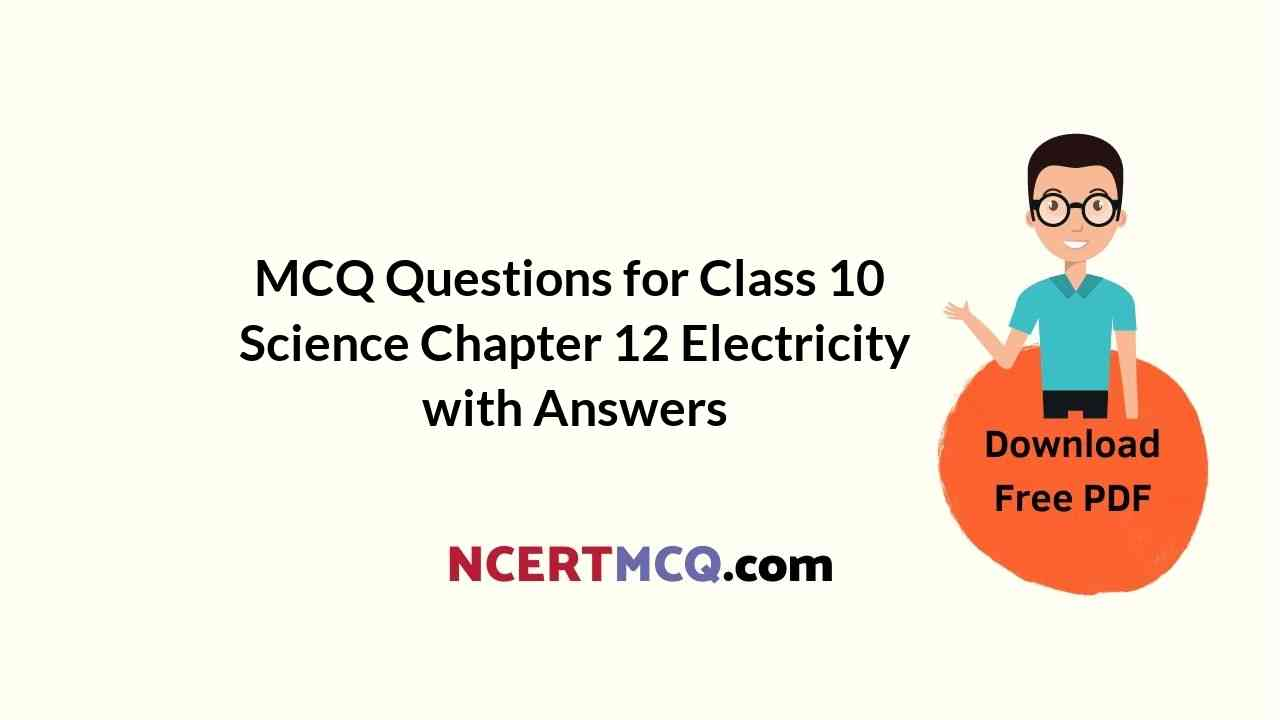Students can also read Online Education MCQ Questions for Class 10 Science Chapter 12 Electricity Questions with Answers hope will definitely help for your board exams. https://ncertmcq.com/mcq-questions-for-class-10-science-with-answers/

## Online Education for Electricity Class 10 MCQs Questions with Answers

Electricity Class 10 MCQ Question 1.
The values of mA and µA are:
(a) 10-6 and 10-9 A respectively
(b) 10-3 and 10-6 A respectively
(c) 10-3 and 10-9 A respectively
(d) 10-6 and 10-3 A respectively
(b) 10-3 A and 10-6 A.

Explanation: An ampere is the SI unit of electric current
1A = 1000mA Or 1mA = $$\frac{1 \mathrm{~A}}{1000}$$ = 103A

∴ 1 µA = 10-3 × 10-3 A = 10-6A

Electricity MCQ Class 10 Question 2.
A cylindrical conductor of Length ‘l’ and uniform area of cross-section ‘A’ has resistance ‘R’. Another conductor of Length 2.5 1 and resistance 0.5 R of the same material has area of cross-section.
(a) 5A
(b) 2.5A
(c) 0.5A
(d) $$\frac{1}{5}$$ A

Class 10 Electricity MCQ Question 3.
Identify the circuit in which the electrical components have been properly connected.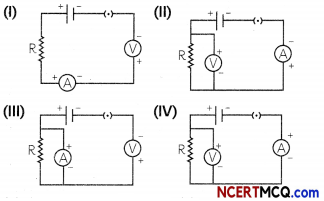(a) (I)
(b) (II)
(c) (III)
(d) (IV)
(b) (II)

Explanation: In figure (I), the voltmeter is connected in series (not parallel). Similarly, in figure (III). the ammeter ¡s in parallel which should be in series. In figure (IV), the negative terminal of battery has been connected to the positive terminal of the ammeter, whereas it should be connected to the negative terminal

Related Theory:
Essential conditions when components connected:

• The voltmeter should be connected in parallel
• The ammeter should be connected in series.
• Positive terminals of the voltmeter arid ammeter should be connected to the positive terminal of the cell and their negative removals should be joined to the negative terminal of the cell

Electricity Class 10 MCQ With Answers Question 4.
If a person has five resistors each of value Ω, then the maximum resistance he can obtain by connecting them ¡s:
(a)1 Ω
(b)5 Ω
(c) 10 Ω
(d) 25 ΩMCQ On Electricity Class 10 Question 5.
What is the minimum resistance which can be made using five resistors, each of $$\frac{1}{5}$$ Ω?
(a) $$\frac{1}{5}$$ Ω
(b) $$\frac{1}{25}$$ Ω
(c) $$\frac{1}{10}$$ Ω
(d) 25 Ω

Class 10 Science Chapter 12 MCQ Question 6.
The resistance of a resistor is reduced to half of its initial value. In doing so, if other parameters of the circuit remain unchanged, the heating effects in the resistor will become:
(a) two times
(b) half
(c) one-fourth
(d) four times
(a) two times

Explanation: Resistance of a resistor R Q New resistance of a resistor Q

All other parameters of the circuit remain unchangedBy applying Joule’s law of heating H = I2Rt

As per Ohms Law V = IR Or I = $$\frac{V}{R}$$Hence, the heating effect in the resistor will become two times if all other parameter of the circuit remain same.

Class 10 Science Chapter 12 MCQ With Answers Question 7.
At the time of short circuit, the electric current in the circuit:
(a) vary continuously
(b) does not change
(c) reduces substantially
(d) increases heavily

MCQ Electricity Class 10 Question 8.
Two bulbs of 100 Ω and 40 Ω are connected in series. The current through the 100 Ω bulb is 1 A. The current through the 40 Ω bulb will be:
(a) 0.4 A
(b) 0.6 A
(c) 0.8 A
(d) 1A
(d) 1 A

Explanation: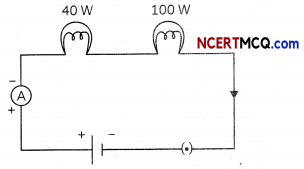In a series combination of resistors (bulbs) the current is the same in every part of the circuit as current does not change in series combination so both the bulbs get equal amount of current Hence the current through the 40 Ωbulb will be 1A.

Ch 12 Science Class 10 MCQ Question 9.
When a 4 V battery is connected across an unknown resistor there is a current of 100 mA in the circuit. The value of the resistance of the resistor is:
(a) 4 Ω
(b) 40 Ω
(c) 400 Ω
(d) 0.4 Ω
(b) 40 Ω
V = IR, V = 4 V,
I = 100 mA = 0.1 A
Hence R = $$\frac{V}{1}=\frac{4}{0.1}$$ Ω = 40 Ω.

Class 10 Science Ch 12 MCQ Question 10.
The equivalent resistance of a series combination of two resistances is X Ohm. If the resistance are of 10 Ohm and 40 Ohm respectively, the value of X will be:
(a) 10 Ohm
(b) 20 Ohm
(c) 50 Ohm
(d) 40 Ohm [Diksha]

Class 10 Physics Electricity MCQ Question 11.
Unit of electric power may also be expressed as:
(a) volt-ampere
(b) kilowatt-hour
(c) watt-second
(d) joule-secondClass 10 Electricity MCQ Questions Question 12.
if the resistance of a certain copper wire is 1 Ω, then the resistance of a similar nichrome wire will be about:
(a) 25 Ω
(b) 30 Ω
(c) 60 Ω
(d) 45 Ω
(c) 60 Ω

Explanation: if the resistance of a certain copper wire is 1 Ω, the resistance of a similar nichrome wire will be about 60 Ω because the resistivity of nichrome is 60 times the resistivity of copper.

Electricity Class 10 MCQ Learn Cbse Question 13.
The resistivity of a certain material is 0.6 Ω m, The material is most likely to be:
(a) an insulator
(b) a superconductor
(c) a conductor
(d) a semiconductor

MCQs On Electricity Class 10 Question 14.
A cell, a resistor, a key, and an ammeter are arranged as shown in the circuit diagrams of the figure. The current recorded in the ammeter will be:(a) Maximum in (I)
(b) Maximum in (II)
(c) Maximum in (III)
(d) The same in all the cases
(d) The same in all the cases

Explanation: In series connections, the order of elements in the circuit will not affect the amount of current flowing in the circuit.

Class 10 Science Electricity MCQ Question 15.
The instrument used for measuring electric current is:
(a) galvanometer
(b) ammeter
(c) voltmeter
(d) potentiometerMCQs Of Electricity Class 10 Question 16.
A student plots V-l graphs for three samples of nichrome wire with resistances R1, R2 and R3. Choose from the following the statement that holds true for this graph.(a) R1 = R2 = R3
(b) R1 > R2 > R3
(c) R3 > R2 > R1
(d) R2 > R1 > R3
(d) R2 > R1 > R3

Explanation: As is clear from the graph, the current for A2 conductor is less than A1 and A1 is Less than A3. We can say IA2 < IA1 < IA3
R2 > R1 > R3 (AS, R = V)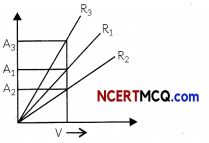Chapter 12 Class 10 Science MCQ Question 17.
The maximum resistance which can be made using four resistors each of resistance $$\frac{1}{2}$$ Ω is:
(a) 2 Ω
(b) 1 Ω
(c) 2.5 Ω
(d) 8 Ω
(a) 2 Ω

Explanation: When a number of resistances are connected in series the resultant resistance is equal to the sum of the individual resistances and is greater than any individual resistance. Four resistors are given: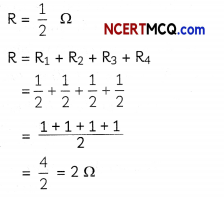Hence, the maximum resistance which can be made using four resistors each of resistance $$\frac{1}{2}$$ Ω is 2 Ω.

Electricity Class 10 MCQs Question 18.
A current of 2 A is drawn by a conductor on applying a potential difference of 6 V across its ends. The number of electrons passing through a cross-section of the conductor and the heat generated in the conductor in 8 seconds is:

 Number of Electrons Passing in 8 s Heat Generated in 8 s (a) 1.6 × 10-20 24J (b) 1020 96J (c) 1.28 × 10-18 3.6 × 106J (d) 1030 96J

Class 10th Science Chapter 12 MCQ Question 19.
The resistivity of four wires A, B, C, and D are of the order of 1012, 10-6, 10-8, and 1014 respectively. !f the wires are of equal lengths and equal areas of cross-section, then select the row containing the correct information:

 Resistance Nature (a) Resistance of C will be the least C is the conductor best of electricity (b) Resistance of A will be the largest A is a good conductor of electricity (c) Resistance of B will be the largest B is a poor conductor of electricity (d) Resistance of D will be the least D is the conductor best of electricity

Question 20.
Given below are four statements on resistance of a conductor. Select the incorrect statements:
(I) If in an electric circuit, the resistance is doubled the voltage also gets doubled.
(II) If in an electric circuit, the resistance is halved the current gets doubled.
(III) Variable resistance is a component used to regulate voltage without changing the current.
(b) Both (I) and (III)

Explanation: According to Ohm’s law, V = IR.
Therefore, l = $$\frac{V}{R}$$.

It means that if resistance R is doubled, the current gets halved, but the voltage remains the same. Similarly, if resistance is halved, currently gets doubled.Question 21.
Given below are four statements about resistivity. Select the incorrect statements:
(I) Resistivity is a characteristic property of the material and varies with temperature.
(II) Resistivity depends on the length and area of the cross-section of the conductor.
(III) Metals and alloys have very low resistivity and are good conductors of electricity.
(IV) Insulators have low resistivity of the order of 1012 to 1017 Ohm.
(a) Both (I) and (II)
(b) Both (I) and (III)
(c) Both (II) and (III)
(d) Both (II) and (iV)

Question 22.
Select the correct statements from the statements given below:
(I) In a parallel circuit the current is constant throughout the electric circuit.
(II) In a parallel circuit the other components keep working even if one component fails.
(III) In a series circuit when one component fails, the circuit is broken and the other components stop working.
(IV) in a series circuit, the current is divided among the electrical gadgets depending upon their resistance.
(a) Both (I) and (II)
(b) Both (II) and (III)
(c) Both (III) and (IV)
(d) (I), (III), and (IV)
(b) Both (II) and (III)

Explanation: In a series circuit, the current is constant through the electric circuit and if one component fails, the other components also stop working. Whereas in a parallel circuit, the current is divided among the different components depending upon their resistance, and if one component fails, the other components keep working.

Question 23.
The unit of potential difference is:
(a) JC
(b) J/C
(c) J
(d) C/J
(b)J/C

Explanation: Potential difference between two points in an electric field of a given charge is defined as the work done in moving a unit positive charge from one point to another. As V = $$\frac{W}{q}$$ , the unit of potential difference is Joule/ Coulomb or J/C.

Question 24.
If both the length of a conductor and its radius is doubled, the ratio of new resistance and original resistance will be:
(a) 1:1
(b) 2:1
(c) 4:1
(d) 1: 2

Question 25.
When a 2 Ω resistor is connected across the terminals of a 6 V battery, the charge (in coulombs) passing through the resistor per second is:
(a) 0.5
(b) 2
(c) 3
(d) 4
(c) 3

Explanation: The charge passing through a resistor per second is the same as the current passing through the resistor since current is defined as the rate of flow of charges. The current flowing is found by using Ohm’s law, V = IR. Therefore, I = V/R = 6/2 = 3A, which is the same as the charge passing in one second.Question 26.
A battery of 6V is connected in series with resistors of 0.1 ohms, 0.2 ohms, 0.3 ohms, 0.4 ohms, and 2 ohms. How much current would flow through the 0.3-ohm resistor?
(a) 0.895A
(b) 1.2 A
(c) 2 A
(d) 3 A

### Assertion Reasoning questions Class 10 Science Chapter 12

For the following questions, two statements are given – One labeled Assertion (A) and the other labeled Reason (R), select the correct answer to these questions from the codes (a), (b), (c), and (d) as given below:
(a) Both (A) and (R) are true and (R) is the correct explanation of the (A).
(b) Both (A) and (R) are true but (R) is not the correct explanation of the (A).
(c) (A) is true but (R) is false.
(d) (A) is false but (R) is true.

Question 27.
Assertion (A): At high temperatures, metal wires have a greater chance of short-circuiting.
Reason (R): Both resistance and resistivity of a material vary with temperature.
(b) Both (A) and (R) are true but (R) is not the correct explanation of the (A).

Explanation: At high temperatures, the free electrons start moving at a greater speed and more heat is evolved. The increased amount of heat melt the insulation of wires and wires have a greater chance of short-circuiting. Sometimes the short circuit may even melt the wire and it may cause fire.

Both resistance and resistivity vary with temperature. When the temperature is increased, the random motion of electrons increases. As a result the number of collisions increases between atoms and electrons.

Question 28.
Assertion (A): When two ends of a metallic wire are connected across the terminals of a cell, then some potential difference is set up between its ends. The direction of electrons are from a positive terminal to negative terminal of the cell.
Reason (R): Electrons are flowing through the conductors from its higher potential to its lower potential end. [Diksha]

Question 29.
Assertion: A fuse wire is always connected in parallel with the mainline.
Reason: If a current larger than the specified value flows through the circuit, fuse wire melts.Question 30.
Assertion(A): Electric appliances with the metallic body have three pin connection, whereas an electric bulb has a two pin connection.
Reason (R): Three pin connection reduce heating of connecting wires. [Diksha]
(c) (A) is true but (R) is false.

Explanation: The three connection of electric appliances with metallic body are live wire, neutraL wire and earth wire. The live and neutraL wire supply electric current to the appliance, whereas the earth wire, which is connected to the metal body of the electric appliance, protects us from electric shock in case we touch the metal body of appliances.

In case of an electric shock, the current passes directly to the earth through the earth wire and we do not feel the electric shock.

On the contrary, three-pin connection do not reduce heating of connecting wires.

Question 31.
Assertion (A): In a purely resistive circuit, the source energy is dissipated in the form of heat.
Reason (R): The potential difference across the ends of a conductor is directly proportional to the current flowing through it.

Question 32.
Assertion (A): Alloys are commonly used in electrical heating devices like electric ion and heater.
Reason (R): Resistivity of an alloy is generally higher than that of its constituent metals but the alloys have low melting points then their constituents, metals.
(a) Both (A) and (R) are true and (R) is correct explanation of the assertion.

Related Theory
Alloys have more resistivity than of its constituent elements because alloys have better properties than that of metals. Resistivity is the property of a material that explains how strongly the material opposes flow of electrons. Higher the resistivity, smaller will be the conductivity i.e. why alloys are used in electrical heating devices.

Metals have a higher melting point as the atoms are arranged in a regular manner and form a strong bond whereas alloys are made up of different metals. The different sizes of atoms in an alloy make their arrangement less regular than a pure metal. This makes the bonds between the atoms weaker and lowers the melting point.

(Competency Based Questions (CBQs))

Question 1.
The following table given below shows the resistivity of three materials X. Y and Z Analyse the table and answer the following questions: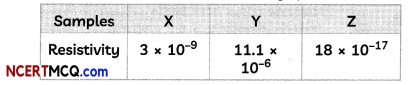(A) Arrange the samples in increasing order of conductivity.
Conductivity is inversely proportional to resistivity so Y < X < Z.

(B) Which of these is the best conductor?
Z is the best insulator as it has the least resistivity.

(C) Which are these is the best insulators?
(a) X
(b)Y
(c) Z
(d) None of these

(D) Electrical resistivity of a given metallic wire depends upon:
(a) Its length
(b) Its thickness
(c) Its shape
(d) Nature of the material
(d) Nature of the material

Explanation: The resistivity of a material depends on the nature and the temperature of the conductor, but not its shape and size.Question 2.
Shivani 8 had studied in her class that an electric bulb consists of a filament made up of a metal having high melting point such as tungsten. Also, the bulbs are filled with a chemically inert gas such as nitrogen or argon to prolong the life of filament.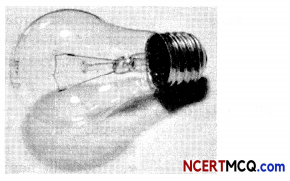A current of 1 A is drawn by a filament of anelectric bulb. The number of electrons passingthrough a cross-section of the filament in 16seconds would be roughly:
(a) 1020
(b) 1016
(c) 1018
(d) 1023
(a) 1020
Explanation: Electric current, 1 = 1 A Time, t = 16 seconds No. of electrons, n = ?

We know that current is the amount of electric charge passing through a given point of conductor in 1 second.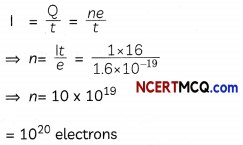The number of eectrons flowing is 1020 electrons.

Question 3.
The following table given below shows the information about two heaters A and B. Analyse the table and answers the following questions: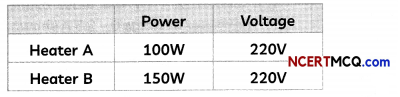(A) Which Heater has higher resistance?
(B) If 1KWh is priced at 30 paise, which heater wilt be costlier if they run for 1 hours each?

Question 4.
Prateek bought a new battery for his Laptopas the earlier battery would not stay charged for more than 5 minutes. He Learnt that a battery consisted of celLs combined together in a particular manner.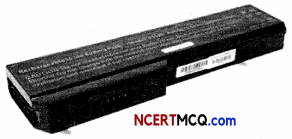The proper representation of series combination of cells obtaining maximum potential is:(a) (I)
(b) (II)
(c) (III)
(d) (IV)

Question 5.
Take a nichrome wire, a torch bulb, a 10 W bulb and an ammeter (0 – 5 A range), a plug key and some connecting wires. Set up the circuit by connecting four dry cells of 1.5 V each in series with the ammeter leaving a gap XV in the circuit, as shown in Fig. below.Complete the circuit by connecting the nichrome wire in the gap XY Plug the key. Note down the ammeter reading. Take out the key from the plug. Replace the nichrome wire with the torch bulb in the circuit and find the current through it by measuring the reading of the ammeter. Now repeat the above step with the 10 Wbu(bin the gap XY

Next, complete an electric circuit consisting of a cell and ammeter, a nichrome wire of Length l [say, marked (1)] and a plug key, as shown in Fig. below.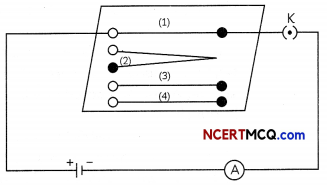Now, plug the key. Note the current in the ammeter. Replace the nichrome wire by another riichrome wire of same thickness but twice the length, that is 21 [marked (2) in the Fig. Note the ammeter reading. Now replace the wire by a thicker nichrome wire, of the same length l [marked (3)]. A thicker wire has a larger cross-sectional area. Again note down the current through the circuit Instead of raking a nichrome wire, connect a copper wire [marked (4) in Fig.] in the circuit Let the wire be of the same length and same area of cross-section as that of the first nichrome wire [marked (1)].
(A) The observation regarding ammeter readings on connecting the different components nichrome wire, torch bulb and a 10 w bulb in the gap XY is tabulated below.
Select the row containing the correct observation and its explanation.

 Observation Explanation (a) Reading of ammeter will be same in all cases Current flowing in a circuit depends only on the potential difference (b) Reading of ammeter will be same in all cases All the components used are good conductors of electric current. (c) Reading of ammeter will be different for different All components resist the flow of electric current by different amounts components (d) Reading of ammeter will be different for different components All components offer equal resistance to the flow of electric current.

(c) Observation: Reading of ammeter will be different for different components: Explanation: All components resist the flow of electric current by different amounts

Explanation: The current is different for different components as certain components offer an easy path for the flow of electric current while the others resist the flow. The motion of electrons through a conductor isretarded by its resistance.

(B) Refer to the second activity and select the incorrect observations:
(I) The ammeter reading will be same in both cases when nichrome wire, marked (1), and copper wire, marked (4), both of length l and area of cross-section A are connected one by one in the circuit.
(II) The ammeter reading will be different in both cases when nichrome wire, marked (1), and copper wire, marked (4), both of same length and cross-section are connected one by one in the circuit.
(III) The current will be double when nichrome wire, marked (2), of length 21 and area of cross-section A is connected as compared to nichrome wire, marked (1) of length l and area of cross-section A.
(IV) The current will be double when nichrome wire, marked (3), of length l and area of cross-section 2A is connected as compared to nichrome wire, marked (1) of length l and area of cross-section A.
(a) Both (I) and (II)
(b) Both (II) and (III)
(c) Both (I) and (IV)
(d) (I), (III) and (IV)
(a) Both (I) and (II)

Explanation: The ammeter reading will be different in both cases when nichrome wire, marked (1), and copper wire, marked (4), both of same length and cross section are connected one by one in the circuit. This is because copper and nichrome are made up of different materials and resistance of a wire depends on the nature of the material.

The current will be double when nichrome wire, marked (3), of length l and area of cross section 2A is connected as compared to nichrome wire, marked (1) of length l and area of cross section A. This is because a thicker wire offers lesser resistance and hence current flowing will be more through a thicker wire as compared to a thinner wire. However, on increasing the length of a conductor to 21. the resistance also becomes double and therefore current decreases to half of its value.

(C) Select the incorrect statement:
(a) The flow of electrons within a conductor is restrained by the attraction of the atoms among which they move.
(b) The electrons are completely free to move within a conductor.
(c) The motion of electrons through a conductor is retarded by its resistance.
(d) A component of a given size that offers a low resistance is a good conductor.

(D) What will be the effect on current flowing in the circuit when length is made double?
(a) Current will remain same
(b) Current will become double
(c) Current will become half
(d) Current will become four times

(E) What will be the least count of an ammeter of range 0 – 1.5 A shown below: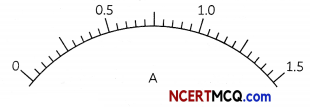(a) 0.1 A
(b) 0.2 A
(c) 0.02 A
(d) 0.05 AQuestion 6.
Wires used for electrical connections have different properties and hence different ratings. So, one must select wires of proper rating to be used for different appliances as different appliances have different requirements.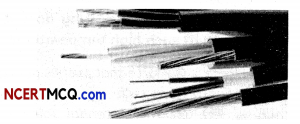Electrical resistivity of a given metallic wire-depends upon:
(a) Its Length
(b) Its thickness
(c) Its shape
(d) Nature of the material

Question 7.
Join three resistors of different values, say, 1 Ω, 2Ω and 3 Ω etc.. and a battery of 6 Vin series. Connect them with a battery, an ammeter and a plug key, as shown in Fig. below.Plug the key. Note the ammeter reading. Change the position of ammeter to anywhere in between the resistors. Note the ammeter reading each time.

In the next activity. insert a voltmeter across the ends X and Y of the series combination of three resistors as shown in Fig. below. Plug the key in the circuit and note the voltmeter reading. It gives the potential difference across the series combination of resistors. Let it be V. Now measure the potential difference across the two terminals of the battery. Compare the two values. Take out the plug key and disconnect the voltmeter. Now insert the voltmeter across the ends X and P of the first resistor, as shown inPlug the key and measure the potential difference across the first resistor. Let it be V1. Similarly, measure the potential difference across the other two resistors, separately. Let these values be V2 and V3, respectively.

(A) Refer to the first activity and circuit diagram at fig. 1. A student recorded his observations in a tabuLar form as shown below. SeLect the row containing correct observations/readings.(a) Position of Ammeter: Between battery and 1 O resistor as shown in figure; Ammeter Beading: 1A; Voltmeter Reading: 6 V.

Explanation: The value of the current in the ammeter is the same and does not depend on its position in the electric circuit.
The total resistance resistance = R1 + R2 + R3 = 6 D.
Therefore, reading of ammeter = I = V / R = 6/6 A = 1 A

(B) Select the correct concLusion based the observations recorded in activity 1 above:
(I) The resistances R1, R2 and R3 are connected in series in the activity.
(II) In a series combination of resistors the current is different in different parts of the circuit.
(III) In a series combination of resistors the current is the same through each resistor.
(IV) In a series combination of resistors the potential difference is the same across each resistor.
(a) Both (I) and (II)
(b) Both (II) and (III)
(C) Both (I) and (III)
(d) Both (II) and (IV)

(C) In the second activity (refer fig. 2), the values of potential difference V, V1, V2 and V3 are:
(a) V = 6 V, V1 = 1 V, V2 = 2 V, V3 = 3 V
(b) V = 6 V, V1 = 1V, V2 = 2 V, V3 = 3 V
(c) V = 6 V, V1 = 1 V, V2 = 2 V, V3 = 3 V
(d) V = 6 V, V1 = 1 V, V2 = 2 V, V3 = 3 V
(d) V = 6 K V1 = 1 V, V2 = 2 K V3 = 3 V

Explanation: The potential difference across the resistors R1, R2 and R3 can be calculated by applying Ohm’s law.
As current I = 1 A, V1 = IR1 = 1 V. Similarly, V2 = 2 V and V3 = 3V.
The potential difference V is equal to the sum of potential differences V1, V2, and V3.

(D) Which of the following relations for V and I is correct for a series combination of three resistors as shown above?
(a) I = I1 + I2 + I3
(b) V = V1 + V2 + V3
(c) $$\frac{1}{V}=\frac{1}{V_{1}}+\frac{1}{V_{2}}+\frac{1}{V_{3}}$$
(d) $$\frac{1}{I}=\frac{1}{I_{1}}+\frac{1}{I_{2}}+\frac{1}{I_{3}}$$

(E) Suppose in the above activity, each resistance is doubled. Select the correct observation:
(a) The total current is doubled
(b) The potential difference is doubled
(c) The total current is halved
(d) The potential difference is halved.

Question 8.
Rahman wanted to change the electric bulb in his study room as he felt that the present bulb was not bright enough for him to study properly. So, he called the electrician. The electrician came and changed the bulb by holding the illuminated bulb with a piece of cloth as the bulb was very hot.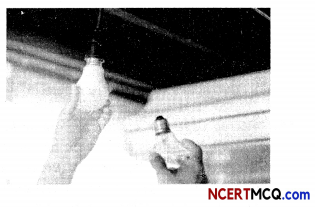The temperature of the filament of bulb is 2700°C when it glows. Why does it not getburnt up at such high temperature?Question 9.
Electric force is the force that pushes apart two like charges, or that pulls together two unlike charges. The electric potential (also called the electric field potential, potential drop, the electrostatic potential) is the amount of work energy needed to move a unit of electric charge (a Coulomb) from a reference point to the specific point in an electric field. The graph of variation of electric potential arising from a point charge Q with distance r from the charge q is shown below: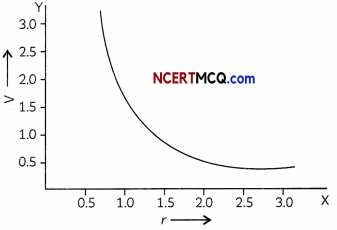Note: Both the charges Q and q are positive charges.
(A) The conclusion that can be made from the above graph between electric potential (V) and distance (r) from the given charge is:
(I) The electric potential decreases as the point charge Q is brought near the given charge q.
(II) The electric potential of a given charge q is inversely proportional to the distance of the point charge from the given charge.
(III) Work is done on a charge to move it closer to another charge of the same sign.
(IV) Less energy is required to bring the point charge Q closer to the given charge q.
Which of the above conclusions are correct?
(a) Both (I) and (II)
(b) Both (II) and (IV)
(c) Both (I) and (III)
(d) Both (II) and (III)

(B) The unit of electric potential is:
(a) J/C
(b) JC
(c) J/s
(d) J/A

(C) The work done in moving a charge of 5 C across two points having a potential difference 12 V is:
(a) 2.4 J
(b) 60 J
(c) 0.42 J
(d) 120 J

(D) The instrument which is used to measure potential difference between two points and type of connection is given below. Select the row containing the correct information.

 Name of instrument Type of connection (a) Ammeter Series (b) Voltmeter Parallel (c) Voltmeter Parallel (d) Voltmeter Series

(E) When 120 J work is done to move 8 C charge from one point to another in an electric field, the potential difference developed between the two points is:
(a) 15 V
(b) 960 J
(c) 960 V
(d) 7.5 V
(D) (c) Name of instrument: Voltmeter; Type of connection: Parallel
Explanation: The instrument used to measure the potential difference between two points is voltmeter and is always connected in parallel across thepoints between which the potential difference is to be measured.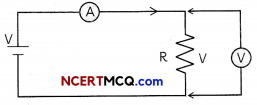Question 10.
Potentiometers, or spots” for short, are used for volume and tone control in electric guitars. They allow us to alter the electrical resistance in a circuit at the turn of a knob. The guitar pickups provide the voltage and current source, while the potentiometers provide the resistance. From Ohm’s Law, we can see how increasing resistance decreases the flow of current through a circuit while decreasing the resistance increases the current flow. If two circuit paths are provided from a common voltage source, more current will flow through the path of least resistance.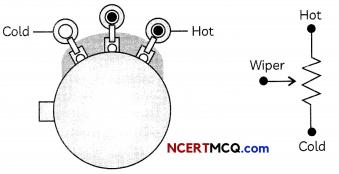We can visualize the operation of a potentiometer from the drawing below.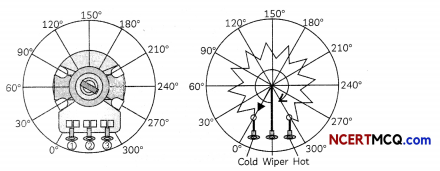Imagine a resistive track connected from terminal 1 to 3 of the pot. Terminal 2 is connected to a wiper that sweeps along the resistive track when the potentiometer shaft is rotated from 0° to 300°. This changes the resistance from terminals 1 to 2 and 2 to 3 simultaneously, while the resistance from terminals 1 to 3 remains the same. As the resistance from terminal 1 to 2 increases, the resistance from terminal 2 to 3 decreases, and vice-versa.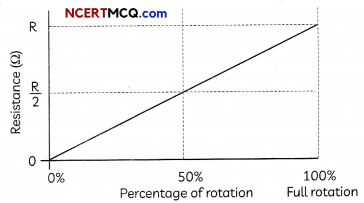(A) When we turn the knob in the potentiometer or pots used in electric guitars, it alters the:
(a) Resistivity of the circuit
(b) Resistance in the circuit
(c) Voltage in the circuit
(d) Effective resistance of the circuit.

(B) Four statements are given below regarding the “cold” and “hot” terminals in the diagram of potentiometer.
Select the correct statements:
(I) The “cold” terminal refers to 0° rotation of the potentiometer shaft and the “hot” terminal refers to 300° rotation of potentiometer shaft.
(II) The “cold” terminal refers to 300° rotation of the potentiometer shaft and the “hot” terminal refers to 150° rotation of potentiometer shaft.
(III) When shaft is at “cold” terminal, resistance in the circuit is maximum, whereas it is minimum when shaft is at “hot” terminal.
(IV) When shaft is at “cold” terminal, resistance in the circuit is minimum, whereas it is maximum when shaft is at “hot” terminal.
(a) Both (I) and (III)
(b) Both (II) and (III)
(c) Both (I) and (IV)
(d) Both (II) and (IV)
(c) Both (I) and (IV)

Explanation: When the potentiometer shaft is turned at 0°, it refers to the “cold” terminal and at this position the resistance is minimum. When the potentiometer shaft is turned at 300°, it refers to the “hot’’ terminal and at this position the resistance is maximum.

(C) When the shaft undergoes 50% rotation, the effective resistance between its terminals is:
(a) R
(b) R/3
(c) 2R
(d) R/2

(D) The device that is used to change resistance in a circuit is:
(a) Rheostat
(b) Fuse
(c) Transformer
(d) Circuit breakers
(a) Rheostat

Explanation: in many cases it may be necessary to increaseor decrease the current in an electric circuit. A component used toregulate current without changing the voltage source is called variabLeresistance. In an electric circuit, a device called rheostat is used tochange the resistance in the circuit.

(E) The table below lists the change in resistance of a wire on changing the physical parameter such as length (l) and area of cross section (A) of the wire. Select the row containing the income Answer:

 Change in Parameter Change in Value of Resistance (a) Length is tripled Resistance becomes three times (b) Area is halved Resistance becomes double (c) Wire is pulled to double its Length Resistance becomes double (d) Diameter is doubted Resistance becomes one fourthQuestion 11.
Ohm law’s application ranges from household appliances like heaters to the high tension wires and massive projects like rockets and spaceships. Ohm’s law is used to maintain the desired voltage drop across the electronic components. Ohm’s law is also used in dc ammeter and other dc shunts to divert the current. We can control the speed of the fans at our homes by moving the regulator to and fro. Here the current flowing through the fan is controlled by regulating the resistance through the regulator. A circular knob on the component can be rotated to achieve a variable resistance on the output terminals. For any specific value of the input, we can calculate the resistance, current and thus power flowing through Ohm’s Law.(A) Refer to the figure-2 above. Select the row containing the correct information: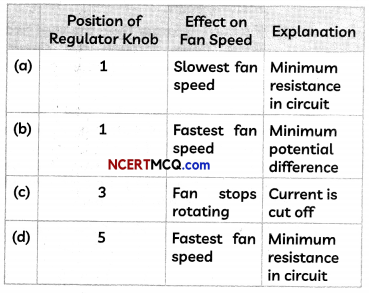(B) The current flowing through a fan is controlled by:
(a) Regulating the voltage through the regulator
(b) Regulating the speed through the regulator
(c) Regulating the resistance through the regulator
(d) Regulating the resistivity through the regulator

(C) A resistance of 5 ohms is further drawn so that its length becomes double. Its resistance will now be:
(a) 20 ohms
(b) 10 ohms
(c) 7.5 ohms
(d) 5 ohms

(D) For a fixed supply voltage the current flowing through a conductor will decrease when
(a) The cross-sectional area of the conductor is increased
(b) The length of the conductor is increased
(c) The length of the conductor is decreased
(d) The cross-sectional area is increased and length is decreased

(E) Observe the V-l graph plotted below and select the incorrect observations:(I) The graph between current (I) and potential difference (V) is a straight line not passing through the origin.
(II) With increase in potential difference, current flowing through the conductor increases.
(III) The slope of the above graph gives the resistivity of the conductor.
(IV) The resistance of the given conductor is 25 Ohm.
(a) Both (I) and (II)
(b) Both (I) and (III)
(c) Both (II) and (III)
(d) Both (111) and (IV)

(C) (a) 20 ohms
Explanation: When a 5 ohm resistance is drawn so that its length becomes double, the area of cross section becomes half. As
R = ρ$$\frac{l}{A}$$ = 5 Ohm, the new resistance will be A
R’ = ρ$$\frac{2l}{A/2}$$ = 4 × ρ$$\frac{l}{A}$$ = 4 × 5 = 20 Ohm.Question 12.
Electric heaters, toasters, geysers, iron and kettles are common appliances used throughout the world. The heaters have a metal coil that has high resistance that permits a certain amount of current to flow through them to provide the required heat as per the Joule’s law of heating. The electric kettle and irons have a lot of resistors in them. The resistors limit the amount of current to flow through them to provide the required amount of heat. The size of resistors used in them is determined by using Ohm’s Law.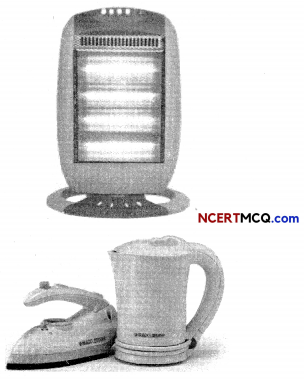(A) According to Joule’s law of heating, the heat produced in a resistor in time t is:
(I) Directly proportional to the square of current for a given resistance
(II) Inversely proportional to the potential difference across the resistance
(III) Directly proportional to resistance for a given current
(IV) Inversely proportional to the time for which the current flows through the resistor.
Select the correct statements.
(a) Both (I) and (II)
(b) Both (II) and (III)
(c) Both (III) and (IV)
(d) Both (I) and (III)
(d) Both (I) and (III)

Explanation: According to Joule’s law of heating, the heat produced in a resistor in time t is given by H = Pt = Vlt = l2Rt ⇒H ∝ l2, H ∝ R, H ∝ t

(B) If the resistance of a resistor is reduced to half of its initial value, potential difference remaining unchanged, the heating effects in the resistor will become:
(a) Half
(b) One fourth
(c) Two times
(d) Four times

(C) Another common application of Joule’s heating is the fuse used in electric circuits. Select the incorrect statement regarding fuse:
(a) It protects circuits and appliances by stopping the flow of any unduly high electric current.
(b) The fuse is placed in parallel with the device.
(c) It consists of a piece of wire made of a metal or an alloy of appropriate melting point.
(d) If a current larger than the specified value flows through the circuit, the fuse wire melts and breaks the circuit.

(D) Consider an electric iron which consumes 1 kW electric power when operated at 220 V. The table below gives the rating of ideal fuse that should be used in this case and the heat generated in the electric iron in 30 seconds.

 Ideal Rating of Fuse (A) Heat Generated in 30 s (J) (a) 4A 3.0 × 104 (b) 10A 1.0 × 104 (c) 5A 3.0 × 104 (d) 5A 3.6 × 106

(E) A resistance of 30 ohm is connected to a 6 V battery. The heat energy in joules generated per minute will be:
(a) 72 J
(b) 144 J
(c) 288 J
(d) 36 J
(A)
(E) (a)72J
Explanation: The heat generated in a resistor is given by Joule’s law of heating,
H = I2Rt.
Here, I = $$\frac{V}{R}=\frac{6}{30}$$ = 0.2A , R = 30 Ohm and t = 60 s.
Therefore, H = (0.2)2 × 30 × 60 = 72 JQuestion 13.
Electrical energy is the energy derived from electric potential energy or kinetic energy of the charged particles. In general, it is referred to as the energy that has been converted from electric potential energy. In many cases it is necessary to calculate the energy usage by an electric device or a collection of devices, such as in a home. The electrical energy (E) used can be reduced either by reducing the time of use or by reducing the power consumption of that appliance or fixture. This will not only reduce the cost, but it will also result in a reduced impact on the environment. Improvements to lighting are some of the fastest ways to reduce the electrical energy used in a home or business. About 20% of a home’s use of energy goes to lighting, while the number for commercial establishments is closer to 40%. A few common electrical appliances and their power ratings are given below:

 Load Types Power Ratings (1) Light bulbs (incandescent) 60W, 80W, 100W (2) Electric (steam) iron 2400W (3) Standing fan 70W (4) Water heater/Kettle 2000W (5) Electric blender 350W (6) Refrigerator 200W (7) Microwave oven 1200W (8) Hand dryer 1800W

(A) Which of the following can be concluded from the table shown above?
(I) The energy consumption of an electrical appliance depends on the power rating and the usage time.
(II) The larger the power rating in the electrical appliance, the lesser is the energy used every second.
(III) The Longer the usage time, the more electrical energy is consumed.
(IV) Appliances that have a heating effect usually have a high power rating and consume more electricity.
(a) Both (I) and (II)
(b) Both (II) and (III)
(c) (I), (II) and (IV)
(d) (I), (III) and (IV)

(B) An electrical water heater/kettle which is marked 2000 W, 220 V means that:
(a) The electric kettle will consume 2000 J of electrical energy every 1 second if it is connected to a 220 V supply.
(b) The electric kettle will consume 7.2 X 106 J of electrical energy every 1 second if it is connected to a 220 V supply.
(c) The electric kettle will consume 440 J of electrical energy every 1 second if it is connected to a 220 V supply.
(d) The electric kettle will consume 3.6 X 106 J of electrical energy every 1 second if it is connected to a 220 V supply.

(C) Students calculated the number of electrical units consumed daily by operating a hand dryer (Power rating 1800 W) for 2 h daily on a supply of 220 V, the total electrical energy consumed in the month of June (in Joule) and the cost of electricity for the month of June if one unit costs Rs. 5.50 and assuming that no other appliance is used.
Select the row containing the correct entries.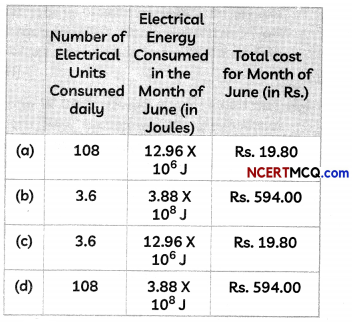(D) The resistance of the refrigerator (Power rating 200 W, 220 V) is:
(a) 44 kilo Ohm
(b) 44 Ohm
(c) 242 Ohm
(d) 484 Ohm

(E) If the microwave oven and the electric blender of power ratings 1200 W and 350 W respectively are both used for 5 hours daily, the electrical energy consumed in a day is:
(a) 6.0 units
(b) 7.75 units
(c) 15.5 units
(d) 232.5 units
(E) (b) 7.75 units
Explanation: The electrical energy consumed in a day is given by E = Pt = 1200 × 5 + 350 × 5 Wh = 7750 Wh = 7.75 kWh or 7.75 units

Very Short Answer Type Questions

Question 1.
Define resistance. Give its S.i. unit.

Question 2.
Define the term electrical resistivity of a material.
Electrical resistivity is defined as the electrical resistance of a conductor having cross¬sectional area 1 m2 and a length 1 m.

Related Theory

• SI unit of electrical resistivity is ohm m (Wm)
• It is a characteristic property of the material and varies with temperature.
• Metals and alloys have very low resistivity but insulators have very high resistivity.

Question 3.
How will the heat produced in a resistor R change if its resistance is reduced to half of its initial value, other parameters of the circuit remain unchanged?

Question 4.
The temperature of the filament of bulb is 2700°C when it glows. Why does it not get burnt up at such high temperature?
Filament of bulb is made using strong metals having high melting points such as Tungsten which has melting point of 3380 °C, which is higher than the temperature at which it glows

Question 5.
Should the resistance of an ammeter below or high? Give reason.Question 6.
Two unequal resistances are connected in parallel. If you are not provided with any other parameters (eg. Numerical value of I and R). What can be said about the voltage drop across the tow resistors?